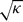Home

# Non-resonant viscous theory for the stability of a fluid-filled gyroscope

## Abstract

In the case of a gyroscope including a cylindrical fluid-filled cavity, the classic Poinsot's coning motion can become unstable. For certain values of the solid inertia ratio, the coning angle opens under the effect of the hydrodynamic torque. The coupled dynamics of such a non-solid system is ruled by four dimensionless numbers: the small viscous parameter ε = Re−1/2 (where Re denotes the Reynolds number), the fluid–solid inertia ratio κ which quantifies the proportion of liquid relative to the total mass of the gyroscope, the solid inertia ratio σ and the aspect ratio h of the cylindrical cavity. The calculation of the hydrodynamic torque on the solid part of the gyroscope requires the preliminary evaluation of the possibly resonant flow inside the cavity. The hydrodynamic scaling used to derive such a flow essentially depends on the relative values of κ and ε. For small values of the ratio/ε (compared to 1), Gans derived an expression of the growth rate of the coning angle. The principles of Gans' approach (Gans, AIAA J., vol. 22, 1984, pp. 1465–1471) are briefly recalled but the details of the whole calculation are not given. At the opposite limit, that is for large values of/ε, the dominating flow is given by a linear inviscid theory. In order to take account of viscous effects, we propose a direct method involving an exhaustive calculation of the flow at order ε. We show that the deviations from Stewartson's inviscid theory (Stewartson, J. Fluid Mech., vol. 5, 1958, p. 577) do not originate from the viscous shear at the walls but rather from the bulk pressure at order ε related to the Ekman suction. Physical contents of Wedemeyer's heuristic theory (Wedemeyer, BRL Report N 1325, 1966) are analysed in the view of our analytical results. The latter are tested numerically in a large range of parameters. Complete Navier–Stokes (NS) equations are solved in the cavity. The hydrodynamic torque obtained by numerical integration of the stress is used as a forcing term in the coupled fluid–solid equations. Numerical results and analytical predictions show a fairly good quantitative agreement.

## References

Hide All
MathJax
MathJax is a JavaScript display engine for mathematics. For more information see http://www.mathjax.org.

# Non-resonant viscous theory for the stability of a fluid-filled gyroscope

## Metrics

### Full text viewsFull text views reflects the number of PDF downloads, PDFs sent to Google Drive, Dropbox and Kindle and HTML full text views.

Total number of HTML views: 0
Total number of PDF views: 0 *Loading metrics...

### Abstract viewsAbstract views reflect the number of visits to the article landing page.

Total abstract views: 0 *Loading metrics...

* Views captured on Cambridge Core between <date>. This data will be updated every 24 hours.

Usage data cannot currently be displayed